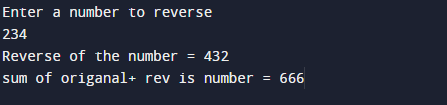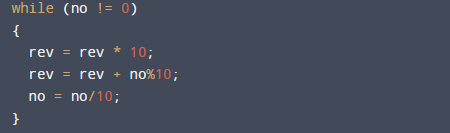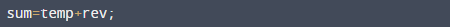# C Program to Add reversed number with Original Number

In this tutorial you will learn about the C Program to Add reversed number with Original Number and its application with practical example.

In this tutorial, we will learn to create a C program that add reversed number with Original number using C programming.

## Prerequisites

Before starting with this tutorial we assume that you are best aware of the following C programming topics:

• Operators.
• loop statements.
• While loop.
• Basic input/output operations.

## C Program to Add reversed number with Original Number.

In this program first of all we reverse a number and that number will be added to original number. For example, user input number 321, the reverse number will be 123.and we add 321 to 123 => 321+123 =  444. Let see the code for that.

## OutputIn the above program, we have first declared and initialized a set variables required in the program.

• no= take number from user.
• rev = it will hold reverse value.
• temp=  it will hold original .
• sum= add original and reverse number.

In the next statement user will be prompted to enter a number into variable no. In our program, we use the modulus (%)operator to obtain digits.
How reversing a number is defined by the following steps:

• 1: START.
•  2:Take a number from user.
•  3: SET temp =no, sum =0.
•  4: REPEAT while (no != 0)
•  5: rev = rev * 10
•  6:rev = rev + no%10
•  7:no = no/10
•  8: sum = no+ temp
•  9: Print Sum
• 10: END.

First of all we take a number from user in variable no. and pass this number to temp variable after that in while loop we reverse the number as show in below image.After reversing a number we add the reverse and temp variable.As you can see how the number is reversing in following steps after the reversing number we add original number sum=temp+rev with reverse number to get required output and print the final value stored in sum variable.

In this tutorial we have learn about the C Program to Add reversed number with Original Number and its application with practical example. I hope you will like this tutorial.